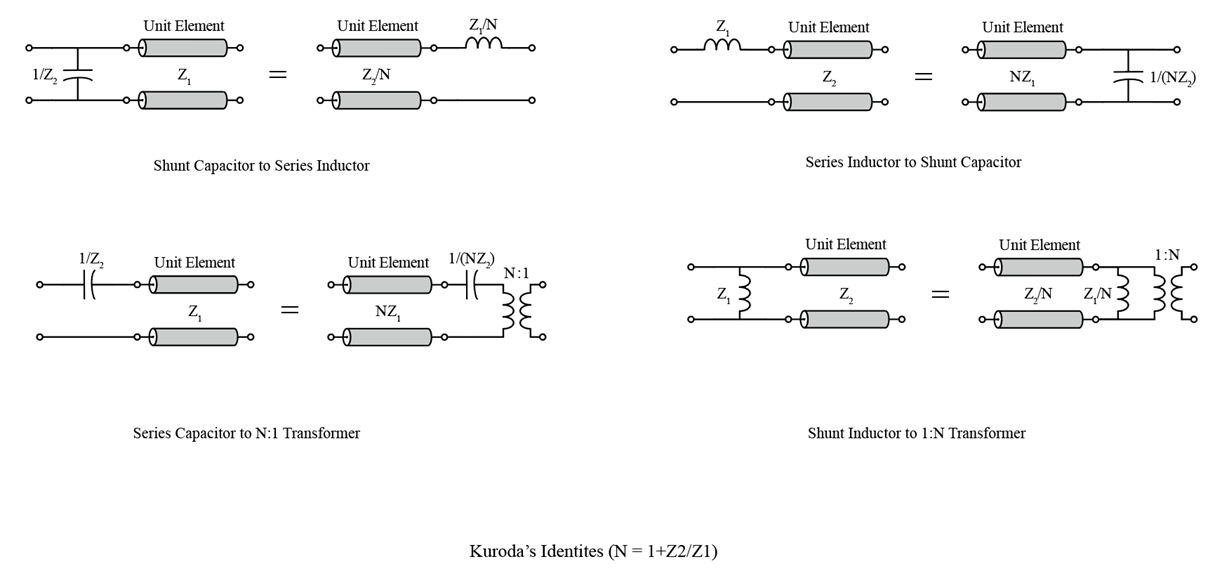# kuroda

Apply Kuroda's transformation based on Kuroda's identities

Since R2021b

## Syntax

``outObj = kuroda(inObj,EL1,EL2)``
``outObj = kuroda(inObj,EL1,EL2,EL3)``

## Description

example

````outObj = kuroda(inObj,EL1,EL2)` applies the suitable Kuroda's identity to the two elements `EL1` and `EL2` in the circuit `inObj`. ```
````outObj = kuroda(inObj,EL1,EL2,EL3)` applies the suitable Kuroda's identity to the of three elements `EL1`, `EL2`, and `EL3`. NoteYou can apply Kuroda's identities only to circuits where all negative terminals of the ports share the same node. ```

## Examples

collapse all

Create a lowpass pi LC ladder object.

```L = 3.18e-8; C = [6.37e-12 6.37e-12]; lpp = lcladder('lowpasspi',L,C);```

Apply Richards' transformation to the LC ladder object.

`r = richards(lpp,1e9)`
```r = circuit: Circuit element ElementNames: {'C_tx' 'L_tx' 'C_1_tx'} Elements: [1x3 txlineElectricalLength] Nodes: [0 1 2 3 4] Name: 'unnamed' NumPorts: 2 Terminals: {'p1+' 'p2+' 'p1-' 'p2-'} ```

Insert a unit element at port 1 of the circuit at the operating frequency of 1 GHz and characteristic impedance of 50 ohms.

`UE = insertUnitElement(r,'C_tx',1,1e9,50)`
```UE = circuit: Circuit element ElementNames: {'C_tx_p1_elem_UE' 'C_tx' 'L_tx' 'C_1_tx'} Elements: [1x4 txlineElectricalLength] Nodes: [0 1 2 3 4 5] Name: 'unnamed' NumPorts: 2 Terminals: {'p1+' 'p2+' 'p1-' 'p2-'} ```

Apply Kuroda's transformation to the two elements `C_tx_p1_elem_UE` and `C_tx`.

`ku = kuroda(UE,'C_tx_p1_elem_UE','C_tx')`
```ku = circuit: Circuit element ElementNames: {'Kuroda2_R2L_of_C_tx_p1_elem_UE' 'Kuroda2_R2L_of_C_tx' 'L_tx' 'C_1_tx'} Elements: [1x4 txlineElectricalLength] Nodes: [0 1 2 3 4 5] Name: 'unnamed' NumPorts: 2 Terminals: {'p1+' 'p2+' 'p1-' 'p2-'} ```

## Input Arguments

collapse all

RF circuit, specified as a `circuit` object. The `circuit` object can have a `txlineElectricalLength` object as one of its elements.

First element in `inObj`, specified as a `txlineElectricalLength` object or a scalar. When you specify the value as scalar, the value refers to an index of element in the circuit. This element must be sequentially connected to the second element specified in the Kuroda's transformation.

Second element in `inObj`, specified as a `txlineElectricalLength` object or a scalar. When you specify the value as scalar, the value refers to an index of element in the circuit. This element must be sequentially connected to the first element specified in the Kuroda's transformation.

Third element in `inObj`, specified as an `nport` object or a scalar. When you specify the value as an `nport` object the value refers to an ideal transformer and when you specify the value as a scalar, the value refers to an index of element in the circuit.

An ideal transformer is implemented by using a 2-port `nport` element with S-parameter data corresponding to an `1:N` or `N:1` ideal transformer. That is, the transformer must be passive, lossless, frequency independent, and with S-parameter data conforming to ```S12 = S21```, and `S12 = N x (1+S11)`, where `N` is the number of turns in a transformer.

This element must be sequentially connected to the first two elements specified in the Kuroda's transformation.

## Output Arguments

collapse all

Circuit element, returned as a `circuit` object.

## Algorithms

collapse all

### Kuroda's Transformation

This figure shows how you can apply Kuroda's transformation or identities to a shunt capacitor, shunt inductor, series capacitor,or series inductor .Pozar, David M. Microwave Engineering. 4th ed. Hoboken, NJ: Wiley, 2012.